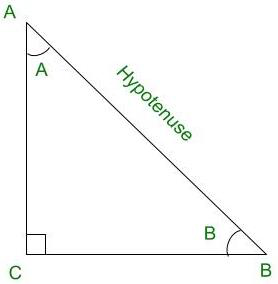GeeksforGeeks App
Open AppBrowser
Continue

# Find the exact value of sin 330°

Trigonometry is the relation between the angles and sides of a right-angled triangle. In a right angles triangle, there are 3 angles of which one angle is a right angle (90°) and other two angles are acute angles and there are 3 sides. The side opposite to the right angle is called Hypotenuse. There are 6 ratios between these sides based on the angle between them and they are called Trigonometric Ratios.

The 6 Trigonometric ratios are:

• Sine (sin)
• Cosine (cos)
• Tangent (tan)
• Cosecant (cosec)
• Secant (sec)
• Cotangent (cot)

All the trigonometric functions are positive in the 1st quadrant. Only sin and cosec are positive in the 2nd Quadrant. Only tan and cot are positive in the 3rd Quadrant and only cos and sec are positive in the 4th Quadrant.Right angled triangle ACB

Sine (sin):

Sine of an angle is defined by the ratio of lengths of sides which is opposite to the angle and the hypotenuse. For the above triangle, the value of angle sine is given for both ∠A and ∠B, the definition for sine angle is the ratio of the perpendicular to its hypotenuse.Cosine (cos):

Cosine of an angle is defined by the ratio of lengths of sides which is adjacent to the angle and the hypotenuse. For the above triangle, the value of the angle cos is given for both ∠A and ∠B, the definition for cos angle is the ratio of the base to its hypotenuse.Tangent (tan):

The tangent of an angle is defined by the ratio of the length of sides which is opposite to the angle and the side which is adjacent to the angle. For the above triangle, the value of the angle tan is given for both ∠A and ∠B, the definition for tan angle is the ratio of the perpendicular to its base.Cosecant (cosec):

The cosecant of an angle is defined by the ratio of the length of the hypotenuse and the side opposite the angle. For the above triangle, the value of the angle cosec is given for both ∠A and ∠B, the definition for cosec angle is the ratio of the hypotenuse to its perpendicular.Secant (sec):

Secant of an angle is defined by the ratio of the length of the hypotenuse and the side and the side adjacent to the angle. For the above triangle, the value of the angle sec is given for both ∠A and ∠B, the definition for sec angle is the ratio of the hypotenuse to its base.Cotangent (cot):

The cotangent of an angle is defined by the ratio of the length of sides that is adjacent to the angle and the side which is opposite to the angle. For the above triangle, the value of the angle cot is given for both ∠A and ∠B, the definition for cot angle is the ratio of the hypotenuse to its base.### Find the exact value of sin (330°)

Solution:

sin is negative in 4th Quadrantsin (360° – θ) = – sin θsin (330°) = sin (360° – 30°)sin (330°) = – sin (30°)sin (330°) = –……. (Since, sin(30°)  =)sin (330°) = – 0.5

Therefore, the exact value of sin (330°) is –.

### Similar Problems

Question 1: Find the exact value of cosec (270°)

Solution:

cosec is positive only in 1st and 2nd Quadrant.

270° does not lies in 1st and 2nd Quadrant.cosec (360° – θ) = – cosec θcosec (270°) = cosec (360° – 90°)cosec (270°) = – cosec (90°)cosec (270°) = – 1                 ……. (Since, cosec(90°) = 1)

Therefore, the exact value of cosec (270°) is – 1 .

Question 2: Find the value of cos (150°)

Solution:

cos is negative in 2nd Quadrantcos (180° – θ) = – cos θcos (150°) = cos (180° – 30°)cos (150°) = – cos (30°)cos (150°) = –……. (Since, cos(30°)  =)

Therefore, the exact value of cos (150°) is –.

Question 3: Find the value of tan (225°)

Solution:

tan is positive in 3rd Quadranttan (180° + θ) = tan θtan (225°) = tan (180° + 45°)tan (225°) = tan (45°)tan (225°) = 1           ……. (Since, tan(45°)  = 1)

Therefore, the value of tan (225°) is 1.

Question 4: Find the value of cosec (225°)

Solution:

cosec is negative in 3rd Quadrant.cosec (180° + θ) = – cosec θcosec (225°) = cosec (180° + 45°)cosec (225°) = – cosec (45°)cosec (225°) = – √2           ……. (Since, cosec(45°) = √2)

Therefore, the value of cosec (225°) is √2.

Question 5: Find the value of cot (315°)

Solution:

cot is negative in 4th Quadrantcot (360° – θ) = – cot θcot (315°) = – cot (360° – 45°)cot (315°) = – cot (45°)cot (315°) = – 1           ……. (Since, cot(45°)  = 1)